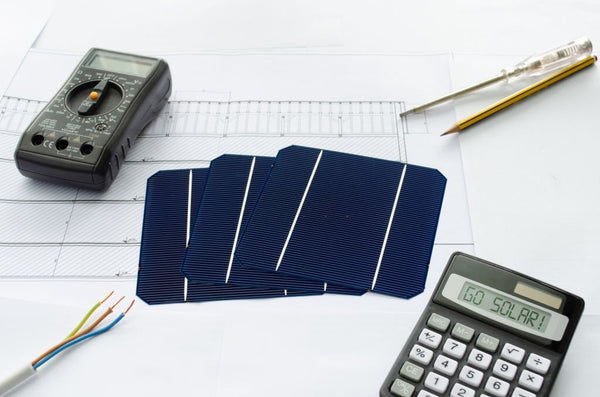Watt Hour To Milliamp Hour Calculator: How To Do It - ShopSolar.com

# Watt-Hour to Milliamp-Hour Calculator

We’ve put together the perfect guide if you’re looking for a watt-hour to milliamp-hour calculator.

Understanding milliamp-hours for your solar setup is important because it shows you how much electrical charge your batteries can hold.

Basically, this calculation helps you understand the capacity of your storage batteries. This means you know how much power you can store to use when the sun is down so your solar setup can power your home 24/7.

Let’s get into what a watt-hour and a milliamp-hour are. Once we understand these terms better, we’ll get into the calculation.

## What Is a Watt-Hour?Watts are a measure of power or energy at any given moment. Watt-hours, on the other hand, measure power over a 60-minute period.

A useful analogy is to think about watts as the speed you’re traveling and watt-hours as the distance you cover.

It’s useful to understand what a watt-hour is because power companies bill you this way. You’ll see that when you receive your bill, you’re billed according to kWh, or kilowatt hours. One kWh is equal to one thousand watt-hours.

In terms of your inverter, the amount of energy it holds is usually displayed in either watt-hours or kilowatt-hours.

These calculations are all extrapolated from Watt’s Law. To understand this better, have a look at our Watt’s law calculator. We also have a watts to watt hours-converter which can give you deeper insight into the topic.

In electrical equations, a watt-hour is expressed as ‘Wh.’ A kilowatt-hour is written as ‘kWh.’ These kinds of watt conversions are common in electrical equations, so it helps to understand them.

## What Is a Milliamp-hour?

A milliamp-hour is 1,000th of an amp hour. Simply put, an amp hour describes how much energy a battery can hold, and how long it can hold it for before it needs to be recharged.

When it comes to your inverter connected to your solar setup, it’s important to know how long it can hold a charge so that you don’t deplete it completely.

When inverter batteries are completely depleted, they degrade faster than they otherwise would.

Milliamp-hours are represented with ‘mAh’ in electrical equations.

You would have seen this being used before on the specs of batteries for electronics, such as your cellphone.## Watt-Hour to Milliamp-Hour Calculator

Thanks to some smart people who came before us, there’s an easy formula we can use to convert watt hours to milliamp hours. In line with our mission to make solar simple, we’re going to explain the equation so that you understand it before you use it.

This is the equation you’ll be using:

mAh = Wh x 1,000 / V

We know that all these symbols can become confusing, so this is how that equation reads in words: milliamp-hours equals watt-hours multiplied by one thousand, divided by voltage.

Let’s go through an example to illustrate how this equation is used.

To determine the milliamp hours, we need to know the watt-hour capacity and voltage of the battery. We use average values here, but you can find the values of your particular inverter in the user’s guide you got with it.

To find the real-world values of your system, have a look at our DC to AC amp calculator to account for the efficiency loss in the inverter.

1,000 Wh is standard, as is a voltage of 48 V.

Your calculation will therefore look like this:

mAh = Wh x 1,000 / V

= 1,000 x 1,000 / 48

= 20,833.33 mAh

## Conclusion

We hope this calculation has helped, but if you’re still a bit confused, have a look at our off-grid solar system packages. These help you avoid having to do calculations, as the components are already grouped to ensure that they work seamlessly together.

We’ve got other tools, like a watt density calculator, that can help you work out all the important factors of your solar system to keep everything running optimally.

Did You Find Our Blog Helpful? Then Consider Checking: